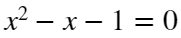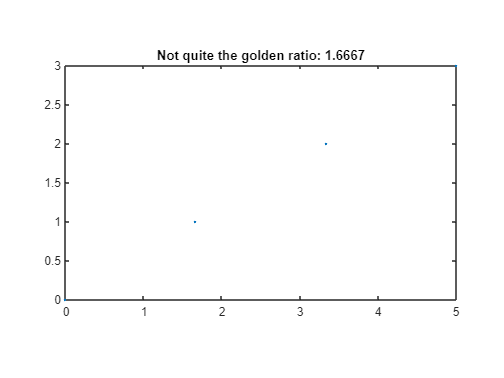## Loren on the Art of MATLABTurn ideas into MATLAB

Note

Loren on the Art of MATLAB has been archived and will not be updated.

# Symmetry, tessellations, golden mean, 17, and patterns

Seventeen? Why 17? Well, as a high school student, I attended HCSSIM, a summer program for students interested in math. There we learned all kinds of math you don't typically learn about until much later in your studies. One of the reference books was Calculus on Manifolds by Michael Spivak. Inside, you learn some of the mysteries of algebra, and, if you read carefully, you will find references to both yellow pigs and the number 17. I leave it as a challenge to you to learn more about either or both if you are interested.As I went to college, the number 17 was a part of my life. Looking through the course catalogue before my first semester, I saw an offering something like "the seventeen regular tilings of the plane", and I signed up. And isn't cool that all of these patterns are displayed in tiles within the Alhambra! I leave you to search the many sites with pictures and drawings of these.
I enjoy the artwork of Rafael Araujo. If you have watched any webinars I have delivered during 2020-2021, you may notice a piece of Araujo's hanging in the background. The basis for much of his work is the golden mean (or golden ratio). Here's a place where you can explore the influence of math on art.

## So what is the golden mean?

It's defined as the solution toAnd the value, typically denoted by the Greek letter
$\varphi \text{\hspace{0.17em}}=\frac{1+\sqrt{5}}{2}\text{\hspace{0.17em}}$
or approximately
phi = (1+sqrt(5))/2
phi = 1.6180
And there are claims that this ratio is universally(?) pleasing. You can see approximations to it show up in everyday life. In the US, we use note cards that are 5x3".
ratio5to3 = 5/3
ratio5to3 = 1.6667
So, close.
plot(0:(5/3):5,0:3,'.')
title("Not quite the golden ratio: " + ratio5to3)
axis equal
axis tightI have written several blogs that show ways to compute Fibonacci numbers, also related to the golden mean. Why? Because ratios of successive Fibonacci numbers converge to the golden mean
$\underset{\mathit{n}\to \infty }{\mathrm{lim}}\frac{{\mathit{F}}_{\mathit{n}+1}}{{\mathit{F}}_{\mathit{n}}}=\varphi \text{\hspace{0.17em}}$

## History

In the 1990s, we held several MATLAB User Conferences. In 1997, I gave a talk on Programming Patterns in MATLAB. I had 17 of them available, but time to only discuss 6 of them. The regular tilings of the plane seemed like a cool way to categorize and clump together some of programming patterns I wanted to talk about. I thought it would be interesting to revisit many of these and see how well they held up over time. So that's my plan for some of the upcoming posts though I feel no compulsion to do all of them or in the order they showed up in my original talk.

## First pattern - data duplication in service of mathematical operations

Steve Eddins wrote two posts on this topic in 2016: one and two. And I wrote one as well, on performance implications.

### Part 1

When I first started at MathWorks (1987), MATLAB had only double matrices and no other data types or dimensions. If I wanted to remove the mean of each column of data in a matrix, I would do something like this.
A(4,4) = 0;
A(:) = randperm(16)
A = 4×4
7 3 11 1 6 10 5 13 12 8 4 2 14 15 16 9
Here I'll calculate the mean of each column.
meanAc = mean(A)
meanAc = 1×4
9.7500 9.0000 9.0000 6.2500
and then I needed to create an array from meanAc that was the same size as A in order to subtract the means. Originally, we did this by matrix multiplication.
Ameans1 = ones(4,1)*meanAc
Ameans1 = 4×4
9.7500 9.0000 9.0000 6.2500 9.7500 9.0000 9.0000 6.2500 9.7500 9.0000 9.0000 6.2500 9.7500 9.0000 9.0000 6.2500
And now I can do the subtraction.
Ameanless1 = A-Ameans1
Ameanless1 = 4×4
-2.7500 -6.0000 2.0000 -5.2500 -3.7500 1.0000 -4.0000 6.7500 2.2500 -1.0000 -5.0000 -4.2500 4.2500 6.0000 7.0000 2.7500
I then met a customer at my first ICASSP conference (in Phoenix, AZ), Tony, and he asked why I was not using indexing instead - because I never thought about it! This is cool because I didn't need to do arithmetic to get my expanded mean matrix.
Ameans2 = meanAc(ones(1,4),:)
Ameans2 = 4×4
9.7500 9.0000 9.0000 6.2500 9.7500 9.0000 9.0000 6.2500 9.7500 9.0000 9.0000 6.2500 9.7500 9.0000 9.0000 6.2500
isequal(Ameans1, Ameans2)
ans = logical 1
That was all well and good - but potentially not so easy to remember each time you might need it.

### Part 2

In 1996, we had heard plenty from customers that we were making something simple a little too difficult. And, we were very close to introducing ND arrays, where we wanted to be able to do similar operations in any chosen dimension(s). So we introduced a new function, repmat.
Now I can find the matrix mean with easier to read code, in my opinion.
Ameanlessr = A - repmat(mean(A),[4,1])
Ameanlessr = 4×4
-2.7500 -6.0000 2.0000 -5.2500 -3.7500 1.0000 -4.0000 6.7500 2.2500 -1.0000 -5.0000 -4.2500 4.2500 6.0000 7.0000 2.7500
isequal(Ameanless1, Ameanlessr)
ans = logical 1

### Part 3

By 2006, we had a lot of evidence that handling really large data was important for many of our customers, and likely to be an increasing demand. Up until then, we always created an intermediate matrix the same size as our original one, A, in order to calculate the result. But this wasn't strictly necessary -- we just need some syntax -- a way to express that all the rows (or columns) would be the same. Now, of course we need a matrix the same size as A for the answer. But how many more arrays of that size did we need along the way? Along came the function, gloriously named bsxfun (standing for binary singleton expansion), and we could perform the computation without fully forming the mxn matrix to subtract from the original.
Ameanlessb = bsxfun(@minus, A, mean(A))
Ameanlessb = 4×4
-2.7500 -6.0000 2.0000 -5.2500 -3.7500 1.0000 -4.0000 6.7500 2.2500 -1.0000 -5.0000 -4.2500 4.2500 6.0000 7.0000 2.7500
isequal(Ameanless1, Ameanlessb)
ans = logical 1

### Part 4

Finally, in 2016, we decided that the meaning was clear even if it wasn't strictly linear algebra, and we now allow many operations to take advantage of implicit expansion of singleton dimensions. What this means for us with this problem is now we can simply say
Ameanless2016 = A - mean(A)
Ameanless2016 = 4×4
-2.7500 -6.0000 2.0000 -5.2500 -3.7500 1.0000 -4.0000 6.7500 2.2500 -1.0000 -5.0000 -4.2500 4.2500 6.0000 7.0000 2.7500
isequal(Ameanless1, Ameanless2016)
ans = logical 1

## Conclusion

I do not expect a Part 5 to come along in 2026, though of course I could be wrong!
Did any of you attend the conferences in 1993, 1995, and 1997? Share your memories here!